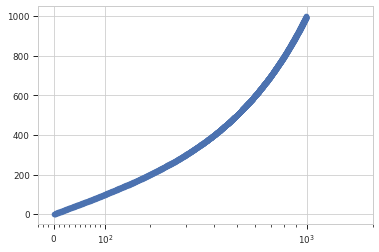# HOWTO: Use the `logicle` scale in other `matplotlib` plots¶

`cytoflow` implements an interesting scaling routine called `logicle`. It’s a biexponential function that is “linear” near zero and transitions smoothly to a logarithmic scale. This is particularly useful for flow cytometry data, which often has data clustered around zero where a log-base-10 scale would introduce aliasing. (See the papers referenced below for rationale and implementation details; `cytoflow` uses the C++ code from the second paper.)

I have been asked several times how to use a `logicle` scale for other `matplotlib` plots. This is difficult because, unlike the `log` scale, `logicle` is parameterized – the precise location of the linear-to-log transition, how many decades of negative data, etc. all need to be specified in order to create a usable scaling function.

However, I recognize that there are occasions when you may want to use a plot that is not baked into `cytoflow`. Here’s a code fragment that should get you pointed in the right direction. However, this is an explicitly unsupported use-case – please don’t file bug reports if you’re having trouble with this:

```import matplotlib.pyplot as plt
import cytoflow as flow

x = range(1, 1000)
y = range(1, 1000)

tube1 = flow.Tube(file = 'data/RFP_Well_A3.fcs',
conditions = {'Dox' : 10.0})
tube2 = flow.Tube(file='data/CFP_Well_A4.fcs',
conditions = {'Dox' : 1.0})

import_op = flow.ImportOp(conditions = {'Dox' : 'float'},
tubes = [tube1, tube2])

ex = import_op.apply()
logicle = flow.utility.scale_factory('logicle', ex, channel = 'V2-A')

# the channel = 'V2-A' parameter in the scale_factory() call tells
# the logicle scale instance to use that channel to estimate its
# parameters.  if you want to use a condition or statistic, say
# condition = ..... or statistic = ..... instead.

plt.scatter(x, y)
ax = plt.gca()
plt.gca().set_xscale('logicle',
**logicle.get_mpl_params(ax.get_xaxis()))
```